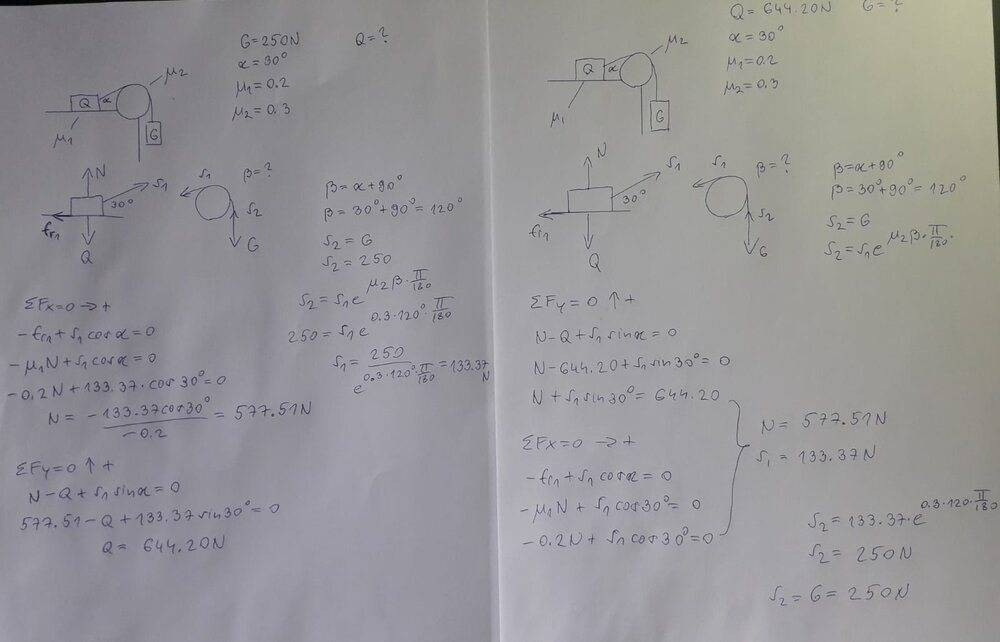# Two blocks connected by a rope over a pulley

In summary, the conversation is about solving a problem involving two masses, G and Q, and their respective positions and angles. The balance is reached when the masses remain in their positions without moving. The problem involves using the Capstan equation, with a given friction coefficient of 0.3 for the pulley, and finding the weight of G. The correct solution is 644.2N, but a mistake was made in the calculation using the incorrect angle of pi/10 instead of pi/5.f

Homework Statement
I needed to find the weight of block Q if given G and vice versa, I already solved it, I just need someone to check it.

For the second block I could choose the weight myself so... I used the same weight I got in the first one for the second so it should be ok, since they match, alternative is I made the same mistake twice.
Relevant Equations
Fx=0
Fy=0Last edited by a moderator:
There are a two things I am not seeing here:
1) A description of what kind of "balance" is supposed to be reached. For example, how would you know if G2 was not massive enough?
2) Solving the weight of G1 given G2 suggests a function. Are you expected to provide that function?

On second look - I was misinterpreting dome of you mu's for M's. So the masses are G and the friction coefficients are ##\mu##'s - and there are no M's.

And the balance is when you get a specific angle ##\alpha##. If ##G_2## is not massive enough, the string angle will be less than ##\alpha##.

You 9's look like 8's or 3's. So what looks like ##\beta = 30° + 30° = 120°## is not.

You state the problem using symbols ##G_1## and ##G_2##, but the symbols in your notes are ##G## and ##Q##. This is important when understanding what you are trying to do - never-the-less, you seem to have you Capstan equation set up correctly.
But, I do not get 133.37 for ##250/{(e^{\pi/10})}##.

The rest looks good to me.

Last edited:
•Yeah I wrote it wrong it aint G1 and G2 but G and Q, I was working on another problem so it sticked with me.

The balance is about all of it remaining in their respective positions they won't move.

Since I made S2=G and G is 250N, then I used it in the Capstan equation and just divided it with
e^(0.3*120*pi/180) since 0.3 is the friction given for the pulley 120 being the angle of the rope contact and and the pi/180 for rads. That is how I got 133.37

•.Scott
I agree with 644.2N.

••